# MATLAB及其信号处理基础

### 原理简介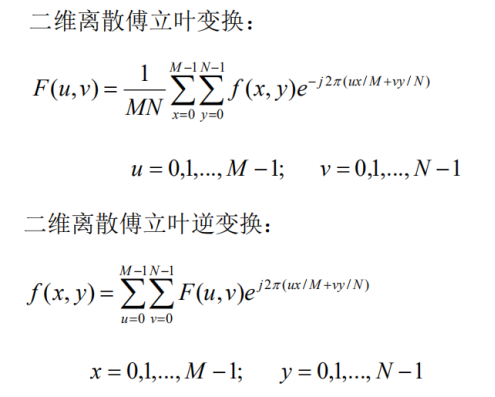二维离散余弦变换对定义：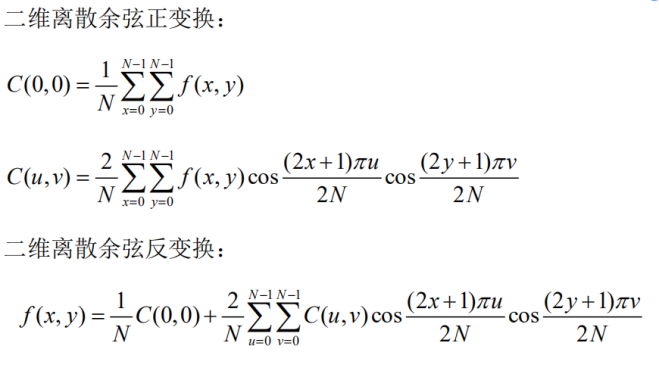### 用离散傅里叶变换分析合成音频文件

1、读取音频文件数据 新建一个 m 文件，另存为 a1.m，输入以下命令：

``clc;clear;l = [1, 40000];[fn, pn] = uigetfile('*.wav', '请选择音频文件');[x, fs] = audioread(strcat(pn, fn),l);len = length(x)``

uigetfile 是文件对话框函数，提供图形界面供用户选择所需文件，返回目标的目录名和文件名。 函数原型：y= audioread (FILE) 功能：读取音频格式文件内容 输入参数：file 表示音频文件名，字符串 返回参数：y 表示音频样点，浮点型

2、一维离散傅立叶变换 新建一个 m 文件，另存为 a2.m，输入以下命令：

``xf = fft(x);f1 = [0:len-1] * fs / len;xff = fftshift(xf);hl = floor(len / 2);f2 = [-hl:hl] * fs / len;``

fft 函数对输入参数进行一维离散傅立叶变换并返回其系数，对应频率从 0 到 fs（采样频率），使用 fftshift 将零频对应系数移至中央。上述代码还计算了离散样点对应的频率值，以便更好地观察频谱。 一维离散傅立叶逆变换 3、新建一个 m 文件，另存为 a3.m，输入以下命令： xsync = ifft(xf); ifft 函数对输入参数进行一维离散傅立叶逆变换并返回其系数。

4、观察结果 新建一个 m 文件，另存为 a4.m，输入以下命令：

``figure;subplot(2, 2, 1);plot(x);title('original audio');subplot(2, 2, 2);plot(xsync);title('synthesize audio');subplot(2, 2, 3);plot(f1, abs(xf));title('fft coef. of audio');subplot(2, 2, 4);plot(f2(1:len), abs(xff));title('fftshift coef. of auio');``

figure(n)表示创建第 n 个图形窗 subplot 是子绘图函数，第一、二个参数指明子图像布局方式，例如，若参数为 2，3 则表示画面共分为 2 行，每行有 3 个子图像。第三个参数表明子图像序号，排序顺序为从左至右，从上至下。 plot 是绘图函数，默认使用方式为 plot(y)，参数 y 是要绘制的数据；如果需要指明图像横轴显示序列，则命令行为 plot(x, y)，默认方式等同于 plot([0…len-1], y)，len 为序列 y 的长度。 用离散傅里叶变换分析合成音频如图所示：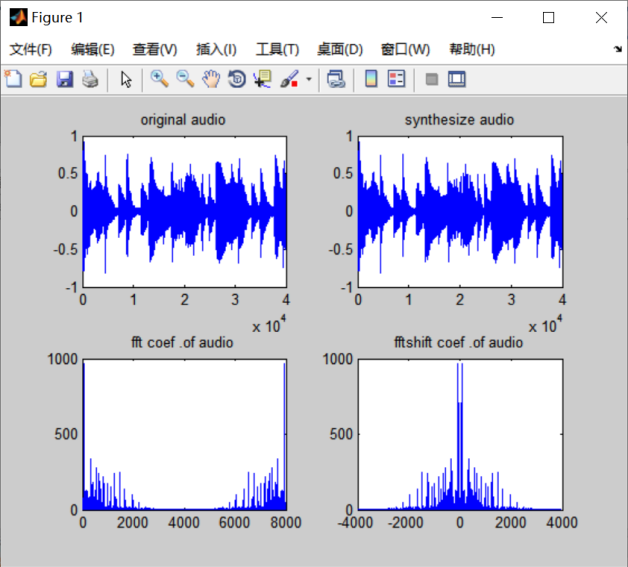### 用离散傅里叶变换分析合成图像文件

1、读取图像文件数据 新建一个 m 文件，另存为 b1.m，输入以下命令：

``[fn, pn] = uigetfile('*.jpg, '请选择图像文件');[x, map] = imread(strcat(pn, fn), jpg');I = rgb2gray(x);``

2、二维离散傅立叶变换 新建一个 m 文件，另存为 b2.m，输入以下命令：

``xf = fft2(I);xff = fftshift(xf);``

fft2 函数对输入参数进行二维离散傅立叶变换并返回其系数，使用 fftshift 将零频对应系数移至中央。

3、二维离散傅立叶逆变换 新建一个 m 文件，另存为 b3.m，输入以下命令：

``xsync = ifft2(xf);``

ifft2 函数对输入参数进行二维离散傅立叶逆变换并返回其系数。 4、观察结果 新建一个 m 文件，另存为b4.m，输入以下命令：

``figure;subplot(2, 2, 1);imshow(x);title('original image');subplot(2, 2, 2);imshow(uint8(abs(xsync)));title('synthesize image');subplot(2, 2, 3);mesh(abs(xf));title('fft coef. of image');subplot(2, 2, 4);mesh(abs(xff));title('fftshift coef. of image');``

imshow 是二维数据绘图函数，mesh 通过三维平面显示数据。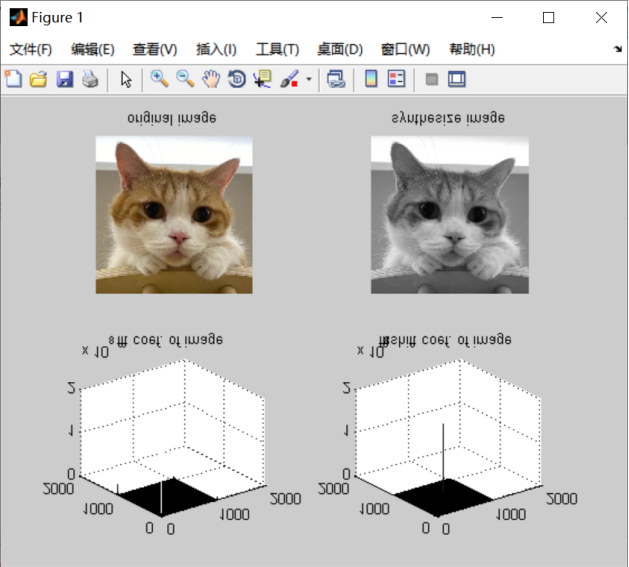### 用离散余弦变换分析合成音频文件

1、一维离散余弦变换 新建一个 m 文件，另存为 c1.m，输入以下命令：

``xf = dct(x);``

dct 函数对输入参数进行一维离散余弦变换并返回其系数，对应频率从 0 到 fs（采样频率）。

2、一维离散余弦逆变换 新建一个 m 文件，另存为 c2.m，输入以下命令：

``xsync = idct(xf);[row,col]=size(x);xff=zeros(row,col);xff(1:row,1:col)=xf(1:row,1:col);y=idct(xff);``

idct 函数对输入参数进行一维离散余弦逆变换并返回其系数。离散余弦变换常用于图像压缩，可以尝试只使用部分系数重构语言，通过观察可发现，原始音频和合成后音频两者差别不大。

3、观察结果 新建一个 m 文件，另存为c3.m，输入以下命令：

``figure;subplot(2, 2, 1);plot(x);title('original audio');subplot(2, 2, 2);plot(xsync);title('synthesize audio');subplot(2, 2, 3);plot(f1, abs(xf));title('fft coef. of audio');subplot(2, 2, 4);plot(f2(1:len), abs(xff));title('fftshift coef. of auio');``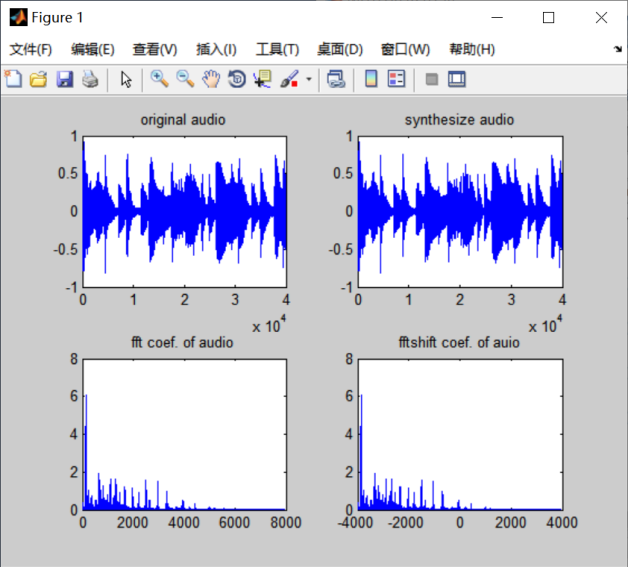### 用离散余弦变换分析合成图像文件

1、二维离散余弦变换 新建一个 m 文件，另存为d1.m，输入以下命令：

``xf = dct2(I);``

dct2 函数对输入参数进行二维离散余弦变换并返回其系数。

2、二维离散余弦逆变换 新建一个 m 文件，另存为d2.m，输入以下命令：

``xsync = uint8(idct2(xf));[row, col] = size(I);lenr = round(row * 4 / 5);lenc = round(col * 4 / 5);xff = zeros(row, col);xff(1:lenr, 1:lenc) = xf(1:lenr, 1:lenc);y = uint8(idct2(xff));``

idct2 函数对输入参数进行二维离散余弦逆变换并返回其系数。可以尝试使用部分系数重构图像，本例中使用了系数矩阵中 4/5 的数据，其它部分置零。为了保证图像能正确显示，使用 uint8 对重构图像原始数据进行了数据类型转换，确保其取值范围在 0 到 255 之间。

3、观察结果 请输入命令显示四个子图，分别是原始图像、使用全部系数恢复的图像，使用部分系数恢复的图像和用三维立体图方式显示系数。 新建一个 m 文件，另存为d3.m，输入以下命令：

``figure;subplot(2, 2, 1);imshow(x);title('original image');subplot(2, 2, 2);imshow(uint8(abs(xsync)));title('synthesize image');subplot(2, 2, 3);imshow(uint8(abs(y)));title('part synthesize image');subplot(2, 2, 4);mesh(abs(xff));title('fftshift coef. of image');``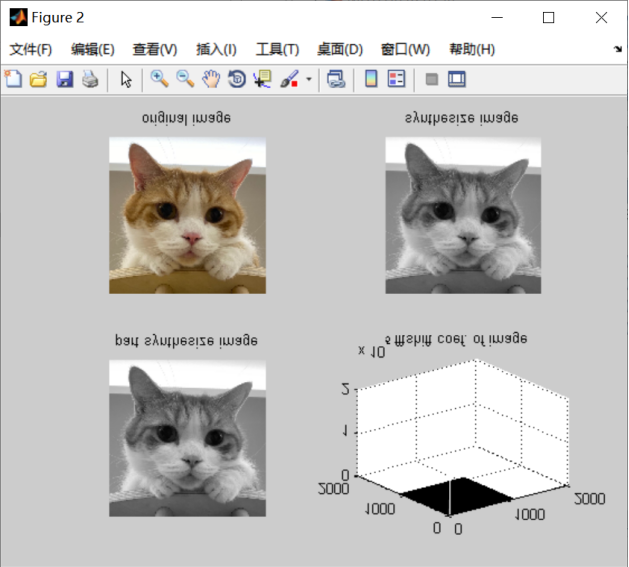### 用离散小波变换分析合成图像文件

1、一维离散小波变换 新建一个 m 文件，另存为 e1.m，输入以下命令：

``[C, L] = wavedec(x, 2, 'db4');``

wavedec 函数对输入参数进行一维离散小波变换并返回其系数 C 和各级系数长度L。第二个参数指明小波变换的级数，第三个参数指明小波变换使用的小波基名称。

2、一维离散小波逆变换 新建一个 m 文件，另存为 e2.m，输入以下命令：

``xsync = waverec(C, L, 'db4');cA2 = appcoef(C, L, 'db4', 2);cD2 = detcoef(C, L, 2);cD1 = detcoef(C, L, 1);``

waverec 函数对输入参数进行一维离散小波逆变换并返回其系数。 appcoef 返回小波系数近似分量，第一个参数 C、第二个参数 L 是 wavedec 的返回参数，为各级小波系数和其长度，第三个参数指明小波基名称，第四个参数指明级。 detcoef 返回小波系数细节分量，第一个参数 C、第二个参数 L 是 wavedec 的返回参数，为各级小波系数和其长度，第三个参数指明级数。

3、新建一个 m 文件，另存为 e3.m，输入以下命令：

``figure;subplot(2, 3, 1);plot(x);title('original audio');subplot(2, 3, 2);plot(xsync);title('synthesize audio');subplot(2, 3, 4);plot(cA2);title('app coef. of audio');subplot(2, 3, 5);plot(cD2);title('det coef. of auio');subplot(2, 3, 6);plot(cD1);title('det coef. of auio');``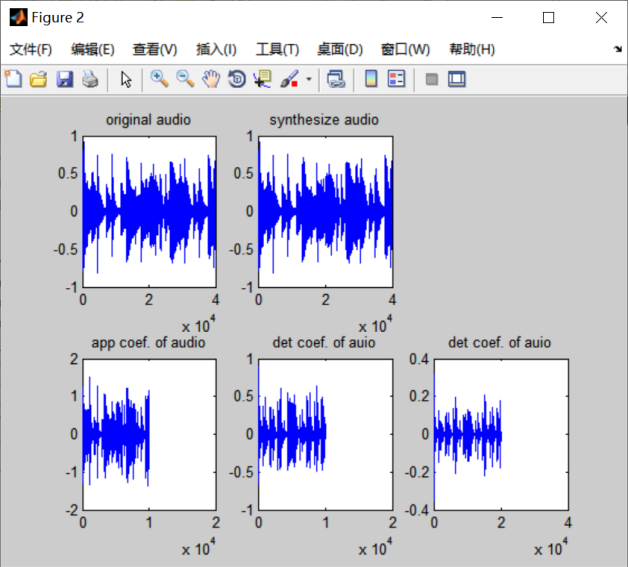### 用离散小波变换分析音频文件

1、二维离散小波变换 新建一个 m 文件，另存为 f1.m，输入以下命令：

``sx = size(I);[cA1, cH1, cV1, cD1] = dwt2(I, 'bior3.7');``

dwt2 函数对输入参数进行二维一级离散小波变换并返回近似分量，水平细节分量，垂直细节分量和对角线细节分量。 如果要对图像进行多级小波分解，使用 wavedec2 函数。 2、二维离散小波逆变换 新建一个 m 文件，另存为f2.m，输入以下命令：

``xsync = uint8(idwt2(cA1, cH1, cV1, cD1, 'bior3.7', sx));A1 = uint8(idwt2(cA1, [], [], [], 'bior3.7', sx));H1 = uint8(idwt2([], cH1, [], [], 'bior3.7', sx));V1 = uint8(idwt2([], [], cV1, [], 'bior3.7', sx));D1 = uint8(idwt2([], [], [], cD1, 'bior3.7', sx));``

idwt2 函数对输入参数进行二维离散小波逆变换并返回其系数。可以尝试仅使用近似分量、水平细节分量、垂直细节分量或对角线细节分量重构图像。 3、观察结果 输入命令显示六个子图，分别是原始图像、使用全部系数恢复的图像、小波系数近似分量、水平细节分量、垂直细节分量和对角线细节分量。 新建一个 m 文件，另存为f3.m，输入以下命令：

``figure;subplot(2, 3, 1);imshow(x);title('original image');subplot(2, 3, 2);imshow(uint8(abs(xsync)));title('synthesize image');subplot(2, 3, 3);mesh(A1);title('app coef. of image ');subplot(2, 3, 4);mesh(H1);title('hor coef. of image ');subplot(2, 3, 5);mesh(V1);title('ver coef. of image ');subplot(2, 3, 6);mesh(D1);title('dia coef. of image ');``